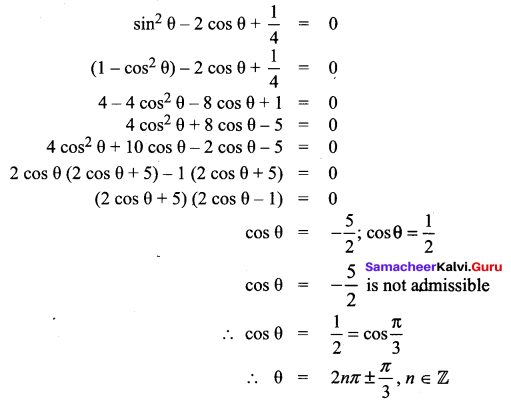# Samacheer Kalvi 11th Maths Solutions Chapter 3 Trigonometry Ex 3.8

## Tamilnadu Samacheer Kalvi 11th Maths Solutions Chapter 3 Trigonometry Ex 3.8

Question 1.
Find the principal solution and general solutions of the following: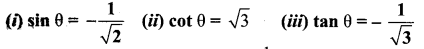Solution: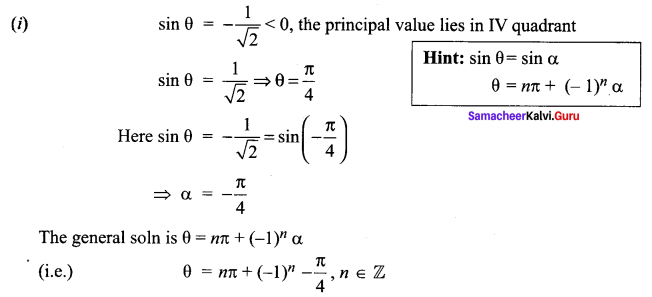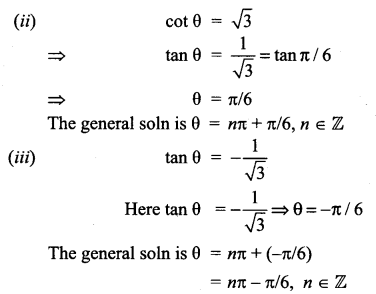Question 2.
Solve the following equations for which solutions lies in the interval 0° < θ < 360°
(i) sin4 = sin2x
(ii) 2 cos2 x + 1 = – 3cos x
(iii) 2 sin2 x + 1 = 3 sin x
(iv) cos 2x = 1 – 3 sin x – 3 sin x
Solution: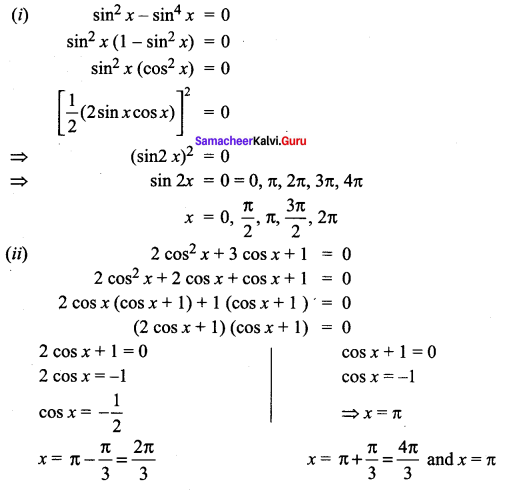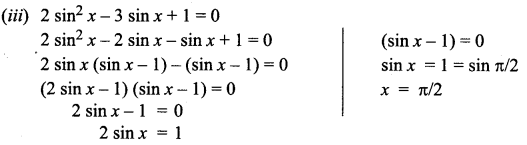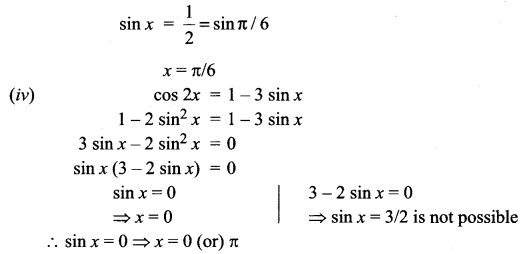Question 3.
Solve the following equations:
(i) sin 5x – sin x = cos 3x
(ii) 2 cos2 θ + 3 sin θ – 3 = 0
(iii) cos θ + cos 3θ = 2 cos 2θ
(iv) sin θ + sin 3θ + sin 5θ = 0
(v) sin 2θ – cos 2θ – sin θ + cos θ = 0
(vi) sin θ + cos θ = $$\sqrt{3}$$
(vii) sin θ + $$\sqrt{3}$$ cos θ = 1
(viii) cot θ + cosec θ = $$\sqrt{3}$$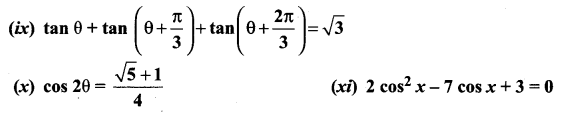Solution: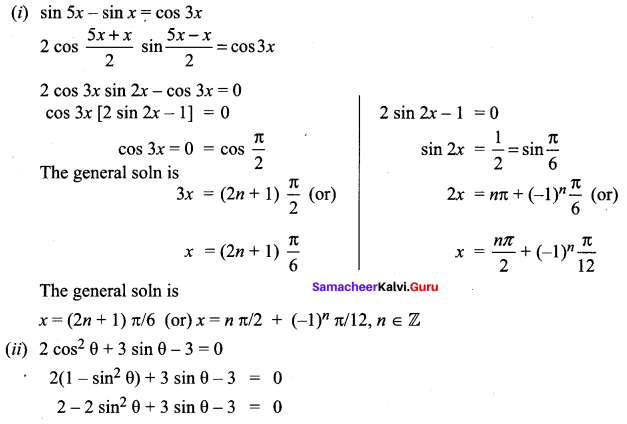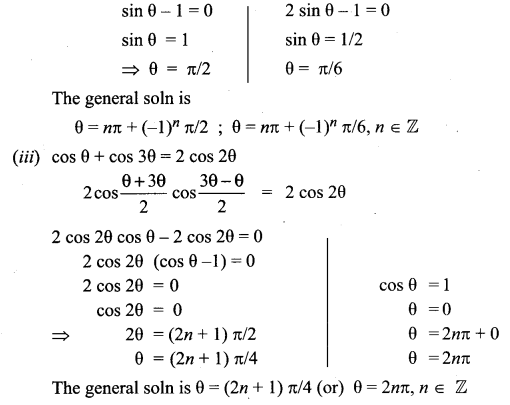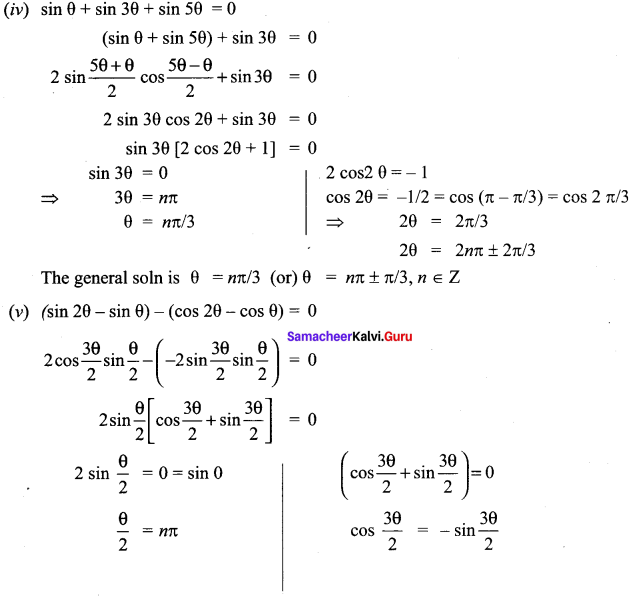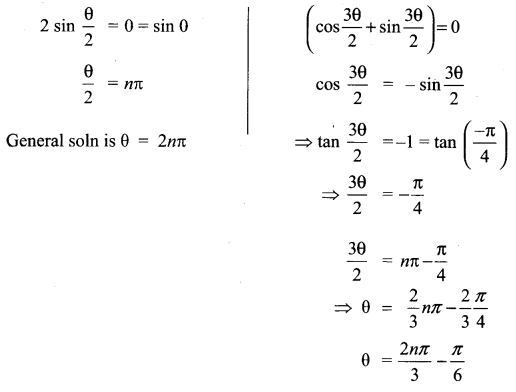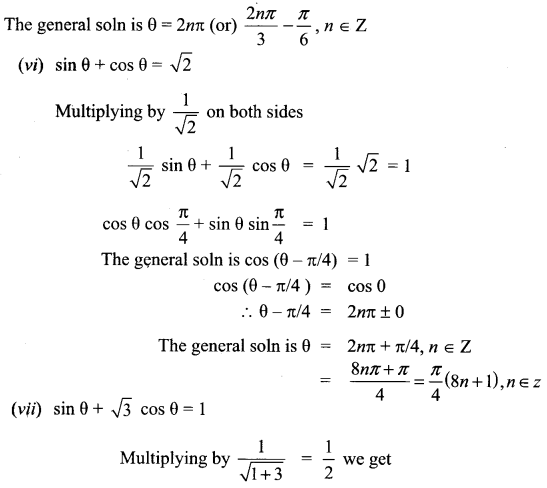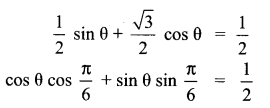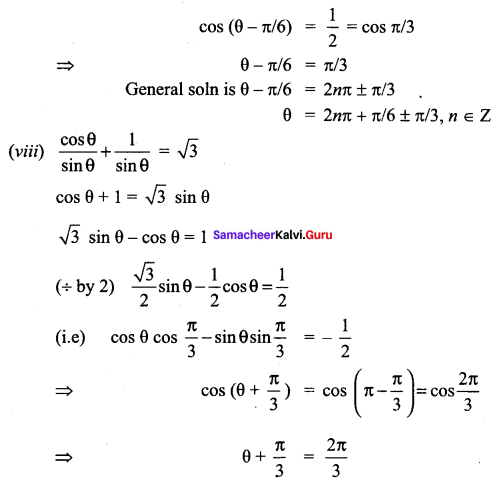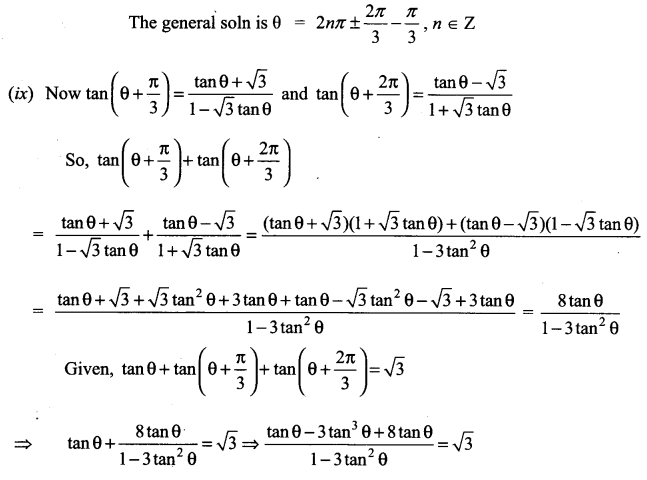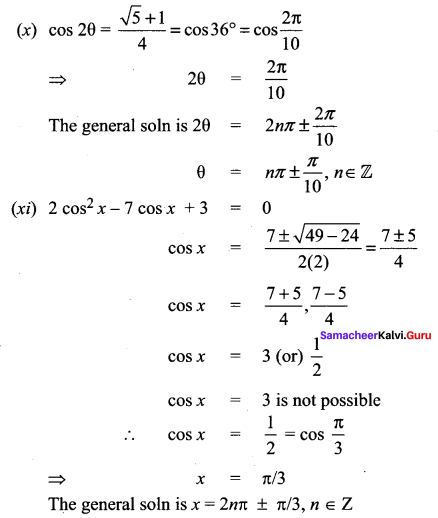### Samacheer Kalvi 11th Maths Solutions Chapter 3 Trigonometry Ex 3.8 Additional Questions

Question 1.
Solve: 2 cos2 θ + 3 sin θ = 0
Solution:
2 cos2 θ + 3 sin θ = 0
⇒ 2 (1 – sin2 θ) + 3 sin θ = 0
⇒ 2 – 2 sin2 θ + 3 sin θ = 0
⇒ -2 sin2 θ + 3 sin θ + 2 = 0
⇒ 2 sin2 θ – 3 sin θ – 2 = 0
⇒ (2 sin θ + 1)(sin θ – 2) = 0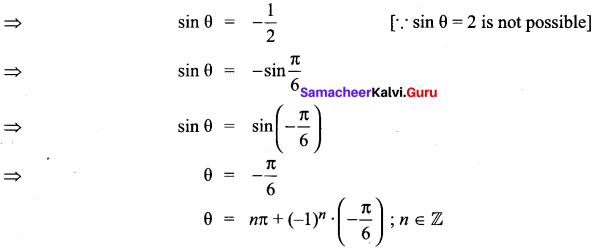Question 2.
Solve: 2 tan θ – cot θ = -1
Solution:
2 tan θ – cot θ = -1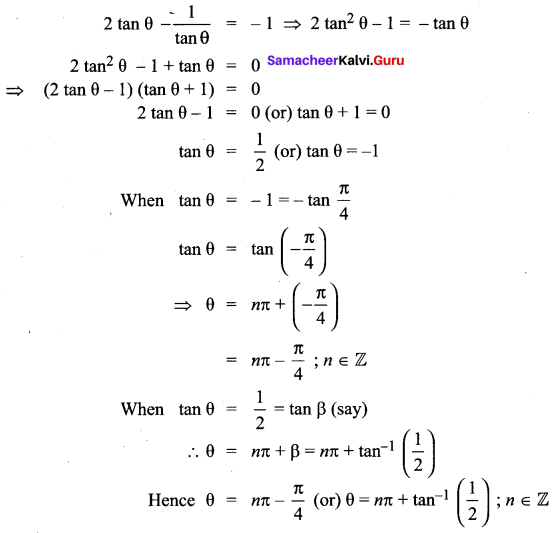Question 3.
Solve: tan2 θ + (1 – $$\sqrt{3}$$) = 0
Solution: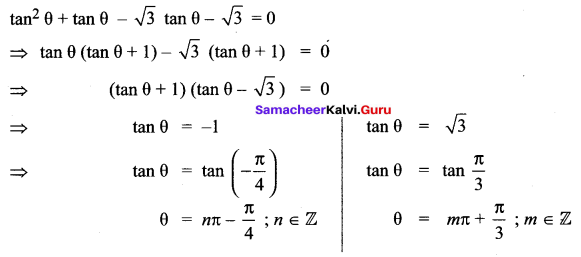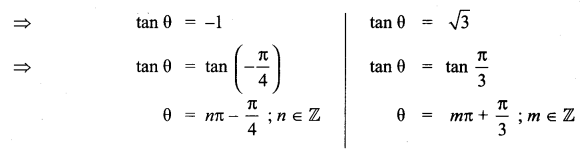Question 4.
Solve: $$\sqrt{3}$$ x + cos x = 2
Solution: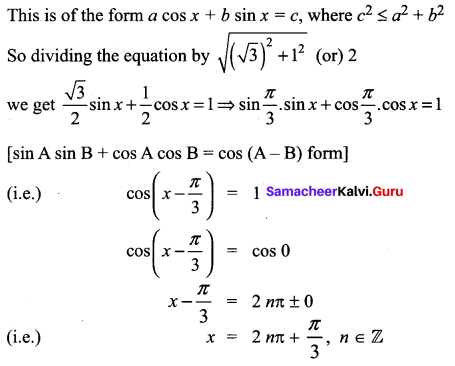Question 5.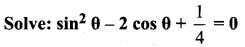Solution: